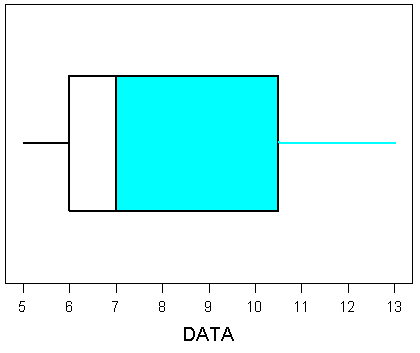Question
173 views

A boxplot for a set of 40 scores is given below.

How many scores are represented in the blue section of the boxplot?help_outlineImage Transcriptionclose6 7 8 9 10 11 12 13 DATA CO LD fullscreen
check_circle

Step 1

The definition of Boxplot was stated below:

Boxplot:

The statistical data is representing in simple way to plot the data based on the five number summary, minimum, first quartile, median, third quartile and maximum.

Step 2

The definition of quartiles was stated below:

Quartiles:

• The first quartile separates the lowest 25% of the observations from the higher 75% of the observations. The first quartile is denoted by Q1.
• The second quartile separates the lower 50% of the observations from the higher 50% of the observations. The second quartile is denoted by Q2.The second quartile is same as median.
• The third quartile separates the lowest 75% of the observations from the higher 25% of the observations. The third quartile is denoted by Q3.
Step 3

The number of scores represented in the blue section of the boxplot is obtained below:

It is given that set of scores are 40.

Here the blue line is above second quartile (Median) so 50% of observations are repre...

### Want to see the full answer?

See Solution

#### Want to see this answer and more?

Solutions are written by subject experts who are available 24/7. Questions are typically answered within 1 hour.*

See Solution
*Response times may vary by subject and question.
Tagged in

### Statistics